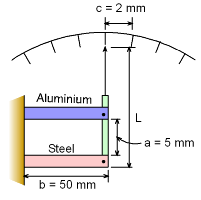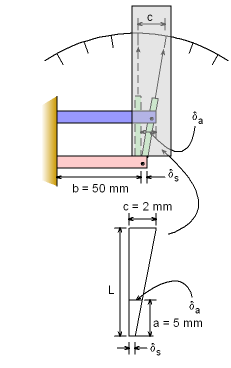Ch 1. Stress and Strain Multimedia Engineering Mechanics NormalStress Shear andBearing Stress NormalStrain Hooke'sLaw ThermalEffects IndeterminateStructures
 Chapter 1. Stress/Strain 2. Torsion 3. Beam Shr/Moment 4. Beam Stresses 5. Beam Deflections 6. Beam-Advanced 7. Stress Analysis 8. Strain Analysis 9. Columns Appendix Basic Math Units Basic Equations Sections Material Properties Structural Shapes Beam Equations Search eBooks Dynamics Fluids Math Mechanics Statics Thermodynamics Author(s): Kurt Gramoll ©Kurt GramollMECHANICS - EXAMPLE ExampleSchematic Diagram of the Thermometer at Room Temperature In a class project a student has decided to make a new type of thermometer. The thermometer assembly consists of two plates, one made of aluminum and the other made of steel. Both plates are the same length, b, at room temperature. When the temperature changes, the plates expand different, causing a pointer to rotate. Each increment on the scale corresponds to a temperature change of 1o F. The distance, c, on the scale is 2 mm which is very small when compared to the length, L, of the indicator, (c << L).The length of each plate, b, is 50 mm. What must be the length of the indicator? The thermal expansion of the indicator can be neglected. The thermal expansion of aluminum, αa, is 13×10-6/oF and that of steel, αs, is 6.5×10-6/oF. SolutionChange of Position of the Indicator with Temperature Change Since each plate is made of different materials their overall length change due to temperature change of 1oF is different.      δa = b αa ΔT          = (50) (13×10-6) (1)          = 6.5×10-4 mm      δs = b αs ΔT          = (50) (6.5×10-6) (1)          = 3.25×10-4 mm Recall, the distance between two consecutive pointer is very small compared to the length of the indicator. So, the length, c, can be assumed to be a straight line. Using similar triangles gives,      (c - δs) / L = (δa - δs) / a      (2 - 3.25×10-4) / L = (6.5×10-4 - 3.25×10-4) / 5      1.9997 / L = 3.25×10-4 / 5      L = 5 (1.9997) / ( 3.25×10-4)      L = 30,760 mm      L = 30.76 m

Practice Homework and Test problems now available in the 'Eng Mechanics' mobile app
Includes over 400 problems with complete detailed solutions.
Available now at the Google Play Store and Apple App Store.